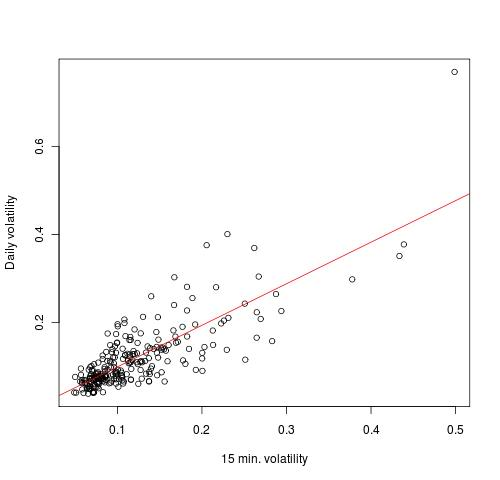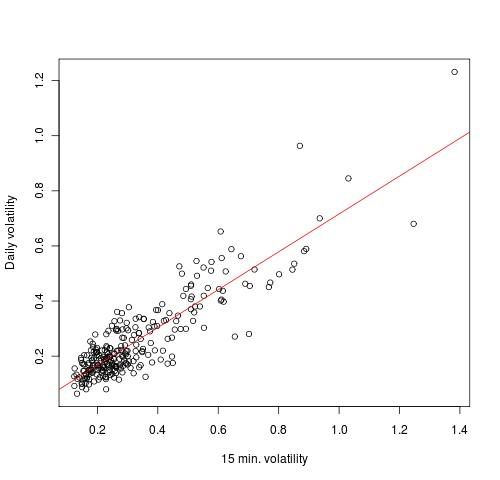Want to share your content on R-bloggers? click here if you have a blog, or here if you don't.

One night I was hooked on this post at elitetrader. I spent couple of hours to find, that a guy is cheating a bit and he didn’t reveal his strategy. Not big deal – free lunch is rear. So, I googled about that guy and one thing catch my attention. In one of his post he mentioned about a stock volatility in the first 15-30 min. and its persistent over whole session. By the way, his new strategy relies on some magic numbers, with I suspect is kind of volatility measurement.Let’s test it.

The rules:
Get volatility of the first 15 or 30 min (1 min. bars used)
Get volatility of the day. (5 min. bars used)
Regress two results and check the correlation.

At the beginning to get volatility I used standard deviation between closing prices. The result was bizarre. So, I used a few neurons in my brain and understood, that time series are definitely non-stationary and I need something else. I decided to use a spread between high of the bar and low of the bar and standard deviation to get volatility.

Results:

Stock1
Correlation 0.798184
Residual Standard Error=0.0474
R-Square=0.6371
F-statistic (df=1, 241)=423.0905
p-value=0

Estimate Std.Err t-value Pr(>|t|)
Intercept   0.0042  0.0064  0.6606   0.5095
X           0.9452  0.0460 20.5692   0.0000Stock2
Correlation 0.8830484
Residual Standard Error=0.0707
R-Square=0.7798
F-statistic (df=1, 262)=927.6893
p-value=0

Estimate Std.Err t-value Pr(>|t|)
Intercept   0.0292  0.0086  3.3704    9e-04
X           0.6868  0.0225 30.4580    0e+00Summary
The correlation between first 15 min. volatility of the day and daily volatility is high.